# 11.3 Systems of nonlinear equations and inequalities: two variables  (Page 5/9)

 Page 5 / 9

## Verbal

Explain whether a system of two nonlinear equations can have exactly two solutions. What about exactly three? If not, explain why not. If so, give an example of such a system, in graph form, and explain why your choice gives two or three answers.

A nonlinear system could be representative of two circles that overlap and intersect in two locations, hence two solutions. A nonlinear system could be representative of a parabola and a circle, where the vertex of the parabola meets the circle and the branches also intersect the circle, hence three solutions.

When graphing an inequality, explain why we only need to test one point to determine whether an entire region is the solution?

When you graph a system of inequalities, will there always be a feasible region? If so, explain why. If not, give an example of a graph of inequalities that does not have a feasible region. Why does it not have a feasible region?

No. There does not need to be a feasible region. Consider a system that is bounded by two parallel lines. One inequality represents the region above the upper line; the other represents the region below the lower line. In this case, no points in the plane are located in both regions; hence there is no feasible region.

If you graph a revenue and cost function, explain how to determine in what regions there is profit.

If you perform your break-even analysis and there is more than one solution, explain how you would determine which x -values are profit and which are not.

Choose any number between each solution and plug into $\text{\hspace{0.17em}}C\left(x\right)\text{\hspace{0.17em}}$ and $\text{\hspace{0.17em}}R\left(x\right).\text{\hspace{0.17em}}$ If $\text{\hspace{0.17em}}C\left(x\right) then there is profit.

## Algebraic

For the following exercises, solve the system of nonlinear equations using substitution.

$\left(0,-3\right),\left(3,0\right)$

$\left(-\frac{3\sqrt{2}}{2},\frac{3\sqrt{2}}{2}\right),\left(\frac{3\sqrt{2}}{2},-\frac{3\sqrt{2}}{2}\right)$

For the following exercises, solve the system of nonlinear equations using elimination.

$\begin{array}{l}\hfill \\ 4{x}^{2}-9{y}^{2}=36\hfill \\ 4{x}^{2}+9{y}^{2}=36\hfill \end{array}$

$\left(-3,0\right),\left(3,0\right)$

$\begin{array}{l}{x}^{2}+{y}^{2}=25\\ {x}^{2}-{y}^{2}=1\end{array}$

$\begin{array}{l}\hfill \\ 2{x}^{2}+4{y}^{2}=4\hfill \\ 2{x}^{2}-4{y}^{2}=25x-10\hfill \end{array}$

$\left(\frac{1}{4},-\frac{\sqrt{62}}{8}\right),\left(\frac{1}{4},\frac{\sqrt{62}}{8}\right)$

$\begin{array}{l}{y}^{2}-{x}^{2}=9\\ 3{x}^{2}+2{y}^{2}=8\end{array}$

$\begin{array}{l}{x}^{2}+{y}^{2}+\frac{1}{16}=2500\\ y=2{x}^{2}\end{array}$

$\left(-\frac{\sqrt{398}}{4},\frac{199}{4}\right),\left(\frac{\sqrt{398}}{4},\frac{199}{4}\right)$

For the following exercises, use any method to solve the system of nonlinear equations.

$\left(0,2\right),\left(1,3\right)$

$\left(-\sqrt{\frac{1}{2}\left(\sqrt{5}-1\right)},\frac{1}{2}\left(1-\sqrt{5}\right)\right),\left(\sqrt{\frac{1}{2}\left(\sqrt{5}-1\right)},\frac{1}{2}\left(1-\sqrt{5}\right)\right)$

$\begin{array}{l}\text{\hspace{0.17em}}\text{\hspace{0.17em}}\text{\hspace{0.17em}}\text{\hspace{0.17em}}\text{\hspace{0.17em}}9{x}^{2}+25{y}^{2}=225\hfill \\ {\left(x-6\right)}^{2}+{y}^{2}=1\hfill \end{array}$

$\left(5,0\right)$

$\left(0,0\right)$

For the following exercises, use any method to solve the nonlinear system.

$\left(3,0\right)$

No Solutions Exist

$\begin{array}{l}\hfill \\ -{x}^{2}+y=2\hfill \\ -4x+y=-1\hfill \end{array}$

No Solutions Exist

$\begin{array}{l}{x}^{2}+{y}^{2}=25\\ {x}^{2}-{y}^{2}=36\end{array}$

$\left(-\frac{\sqrt{2}}{2},-\frac{\sqrt{2}}{2}\right),\left(-\frac{\sqrt{2}}{2},\frac{\sqrt{2}}{2}\right),\left(\frac{\sqrt{2}}{2},-\frac{\sqrt{2}}{2}\right),\left(\frac{\sqrt{2}}{2},\frac{\sqrt{2}}{2}\right)$

$\left(2,0\right)$

$\left(-\sqrt{7},-3\right),\left(-\sqrt{7},3\right),\left(\sqrt{7},-3\right),\left(\sqrt{7},3\right)$

$\left(-\sqrt{\frac{1}{2}\left(\sqrt{73}-5\right)},\frac{1}{2}\left(7-\sqrt{73}\right)\right),\left(\sqrt{\frac{1}{2}\left(\sqrt{73}-5\right)},\frac{1}{2}\left(7-\sqrt{73}\right)\right)$

## Graphical

For the following exercises, graph the inequality.

${x}^{2}+y<9$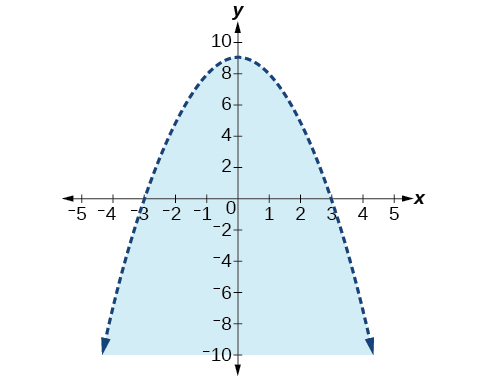${x}^{2}+{y}^{2}<4$

For the following exercises, graph the system of inequalities. Label all points of intersection.

$\begin{array}{l}{x}^{2}+y<1\\ y>2x\end{array}$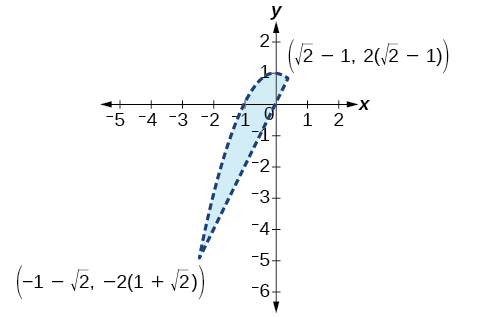$\begin{array}{l}{x}^{2}+y<-5\\ y>5x+10\end{array}$

$\begin{array}{l}{x}^{2}+{y}^{2}<25\\ 3{x}^{2}-{y}^{2}>12\end{array}$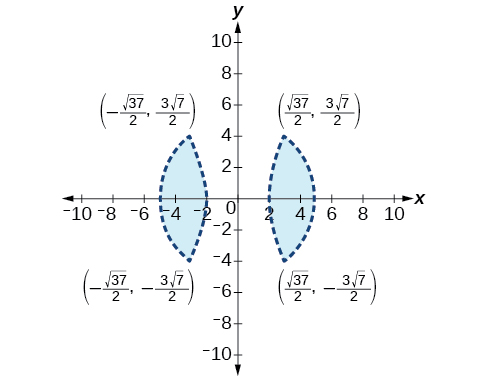$\begin{array}{l}{x}^{2}-{y}^{2}>-4\\ {x}^{2}+{y}^{2}<12\end{array}$

$\begin{array}{l}{x}^{2}+3{y}^{2}>16\\ 3{x}^{2}-{y}^{2}<1\end{array}$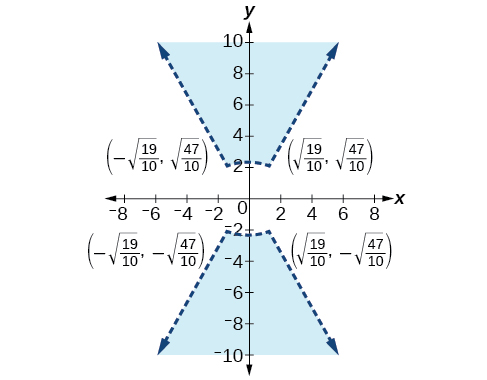## Extensions

For the following exercises, graph the inequality.

$\begin{array}{l}\hfill \\ y\ge {e}^{x}\hfill \\ y\le \mathrm{ln}\left(x\right)+5\hfill \end{array}$

$\begin{array}{l}y\le -\mathrm{log}\left(x\right)\\ y\le {e}^{x}\end{array}$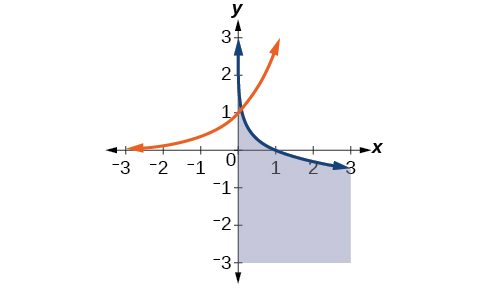For the following exercises, find the solutions to the nonlinear equations with two variables.

$\begin{array}{l}\frac{4}{{x}^{2}}+\frac{1}{{y}^{2}}=24\\ \frac{5}{{x}^{2}}-\frac{2}{{y}^{2}}+4=0\end{array}$

$\begin{array}{c}\frac{6}{{x}^{2}}-\frac{1}{{y}^{2}}=8\\ \frac{1}{{x}^{2}}-\frac{6}{{y}^{2}}=\frac{1}{8}\end{array}$

$\left(-2\sqrt{\frac{70}{383}},-2\sqrt{\frac{35}{29}}\right),\left(-2\sqrt{\frac{70}{383}},2\sqrt{\frac{35}{29}}\right),\left(2\sqrt{\frac{70}{383}},-2\sqrt{\frac{35}{29}}\right),\left(2\sqrt{\frac{70}{383}},2\sqrt{\frac{35}{29}}\right)$

No Solution Exists

## Technology

For the following exercises, solve the system of inequalities. Use a calculator to graph the system to confirm the answer.

$\begin{array}{l}xy<1\\ y>\sqrt{x}\end{array}$

$x=0,y>0\text{\hspace{0.17em}}$ and $0

$\begin{array}{l}{x}^{2}+y<3\\ y>2x\end{array}$

## Real-world applications

For the following exercises, construct a system of nonlinear equations to describe the given behavior, then solve for the requested solutions.

Two numbers add up to 300. One number is twice the square of the other number. What are the numbers?

12, 288

The squares of two numbers add to 360. The second number is half the value of the first number squared. What are the numbers?

A laptop company has discovered their cost and revenue functions for each day: $\text{\hspace{0.17em}}C\left(x\right)=3{x}^{2}-10x+200\text{\hspace{0.17em}}$ and $\text{\hspace{0.17em}}R\left(x\right)=-2{x}^{2}+100x+50.\text{\hspace{0.17em}}$ If they want to make a profit, what is the range of laptops per day that they should produce? Round to the nearest number which would generate profit.

2–20 computers

A cell phone company has the following cost and revenue functions: $\text{\hspace{0.17em}}C\left(x\right)=8{x}^{2}-600x+21,500\text{\hspace{0.17em}}$ and $\text{\hspace{0.17em}}R\left(x\right)=-3{x}^{2}+480x.\text{\hspace{0.17em}}$ What is the range of cell phones they should produce each day so there is profit? Round to the nearest number that generates profit.

A laser rangefinder is locked on a comet approaching Earth. The distance g(x), in kilometers, of the comet after x days, for x in the interval 0 to 30 days, is given by g(x)=250,000csc(π30x). Graph g(x) on the interval [0, 35]. Evaluate g(5)  and interpret the information. What is the minimum distance between the comet and Earth? When does this occur? To which constant in the equation does this correspond? Find and discuss the meaning of any vertical asymptotes.
The sequence is {1,-1,1-1.....} has
how can we solve this problem
Sin(A+B) = sinBcosA+cosBsinA
Prove it
Eseka
Eseka
hi
Joel
June needs 45 gallons of punch. 2 different coolers. Bigger cooler is 5 times as large as smaller cooler. How many gallons in each cooler?
7.5 and 37.5
Nando
find the sum of 28th term of the AP 3+10+17+---------
I think you should say "28 terms" instead of "28th term"
Vedant
the 28th term is 175
Nando
192
Kenneth
if sequence sn is a such that sn>0 for all n and lim sn=0than prove that lim (s1 s2............ sn) ke hole power n =n
write down the polynomial function with root 1/3,2,-3 with solution
if A and B are subspaces of V prove that (A+B)/B=A/(A-B)
write down the value of each of the following in surd form a)cos(-65°) b)sin(-180°)c)tan(225°)d)tan(135°)
Prove that (sinA/1-cosA - 1-cosA/sinA) (cosA/1-sinA - 1-sinA/cosA) = 4
what is the answer to dividing negative index
In a triangle ABC prove that. (b+c)cosA+(c+a)cosB+(a+b)cisC=a+b+c.
give me the waec 2019 questionsByByByByBy